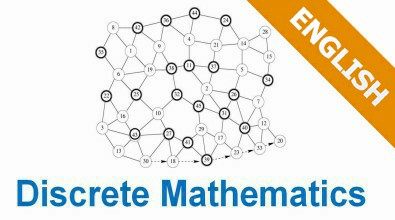# Discrete Mathematics

Enrollment in this course is by invitation onlyWelcome to this course!

A course of Discrete Mathematics has more than one objective. To deal with a problem, students need to study a  congregation of all mathematics information and how to process them by reasoning or inductive reasoning in order to give out the results handling the problems. More importantly, with this course, students need to know how to think logically and mathematically. To achieve these goals, there are a lot of mathematical skills to be gained. Five significant skills are nested in learning Maths: mathematical reasoning, combination analysis, discrete structure, algorithmic thinking, application, and modeling. A successful discrete math course should carefully mix and balance all these five topics.

1 - Mathematical ReasoningStudents must understand mathematical reasoning in order to read, understand, and construct mathematical reasoning. This course begins with mathematical logic problems and it is the basis for the next knowledge, for problems to be proved later. Mathematical induction is emphasized through many different types and it shows that it is a valid proof.

2 - Combination Analysis: An important problem-solving skill is the ability to enumerate objects. This course gives an introduction to the basic technique of counting applied in the complexity analysis techniques of the later algorithm for students studying computer science.

3 - Discrete Structure: A course in Discrete Mathematics. Thus, students will know how to work with discrete structures, which are the abstract mathematical structures used to represent discrete objects and relationships among objects. Discrete structures include sets, permutations, relations, graphs, trees, and finite elements.

4 - Algorithmic Thinking: After an algorithm has been described, a computer program can be constructed to execute it. The mathematical part of this operation, which includes algorithm specifications  and the verification that it works properly, and the analysis of computer memory and the necessary time to execute it, all are identified in this course.

5 - Application and Modeling: Discrete Mathematics has applications for almost every imaginable area and has been studied. There are many computer science applications in this course, as well as applications for different areas such as chemistry, botany, zoology, linguistics, geography, business, and Internet.

Once again, welcome you and wish you much knowledge and many skills from this course!

Course code:

Course name:

Discrete Mathematics

Credit:

3

Estimated hours:

45 hours

Course objectives:

After finishing the course, students can gain:

A. In terms of knowledge

• Know the basic concept of presenting logic and logical predicates
• Know the basic concept of sets, functions, sequences and summations
• Be aware of inductive method
• Basic concepts of algorithms, recursive algorithms
• Know the basic concept of numeral system and recurrence formula
• Know the basic concept of relations and their properties
• Know the basic concept of graphs, key terms, graph types, graph presentation and its connected properties
• Be aware of Euler and Hamilton paths to apply to the shortest path problem
• Know the basic concept of trees and their applications

B. In terms of skills

• Students can manipulate logical expressions which lead to equivalent logical expressions.
• Students can demonstrate simple mathematical propositions which include applying the principles of induction
• Solve problems in the numeral system to apply into algorithm analysis
• Use relation structures
• Solve problems related to paths and graph tree problems

C. Others

After learning this course, students can apply discrete mathematics to deal with computer science-related problems.

Course Structure:

Part 1: Basic knowledge: Logic and Proofs

- Introduction to logical proposition, conditional statement, converse statement and biconditional statement as well as introduction to equivalent proposition, predicate and quantifier language, nested quantifier.

Part 2: Basic structures of Sets, Functions, Sequences and Summations

- Introduction to some concepts of sets, subsets, set operations, functions, sequences and summations.

Part 3: Basic knowledge: Algorithms and Integers

- Introduction to the basic knowledge of algorithms as well as the increase of functions, operations related to integers such as finding the greatest common divisors (GCD) or lowest common multiples (LCM), in which Euclid algorithms are introduced.

Part 4: Mathematical Reasoning, Inductions and Recursions

- Introduction to the principles of inductions as well as recursive formula, recursive algorithms for basic problems to complex ones.

Part 5: Counting elements

- Introduction to counting principles, including two basic problems which are principles with order - permutation formula and without order - combination formula

Part 6: Recursion Relations

- Introduction to recurrence formula and its examples.

Part 7: Relations and their properties

- Introduction to relations and their properties

Part 8: Graphs

- Basic concepts related to graphs such as key terms, some special graph types, graph presentation, isomorphic graphs, connected properties of graphs, Euler và Hamilton paths and applying to the shortest way problem.

Part 9: Trees

- Introduction to graph trees, including the concept of trees and the applications of binary trees

(The mark is rounded to the nearest tenth)

1. On-going Assessment:

• Ask questions, accounting for 15% of total points
• Quiz, accounting for 20% of total points
• Assignment, accounting for 40% of total points

2. Final Examination

• Oral exam, accounting for 25% of total points.

Conditions to participate in the final exam:

• Ask mentor  at least 8 accepted questions
• Obtain 100% points of all quizzes
• Assignment points must be above 0

Passing conditions:

• The final exam mark must be >=4 (out of 10)
• Total score >= 5 (out of 10)

Course Designer

Phung Duy Khuong

LEARNING SOURCES

Below is the list of all free massive open online learning sources (MOOC) used for this course by FUNiX: Ant0nMath, Arnaldo Pedro Figueira Figueira, CourseHack, Daniel Krashen, Derek Banas, Dragonfly Statistics, GVSUmath, James Olsen, Jang Soo Kim, Jason Pallett, Kailee Gray, Karen Daly, Khan Academy, nagerhard, patrickJMT, Professor Heather Pierce, TheTrevTutor.

All open learning sources used in FUNiX training program are public, constantly updated and freely used. FUNiX is always willing to receive and discuss any comments, suggestions and responses related to our learning sources via email program@funix.edu.vn.

1. Course Number